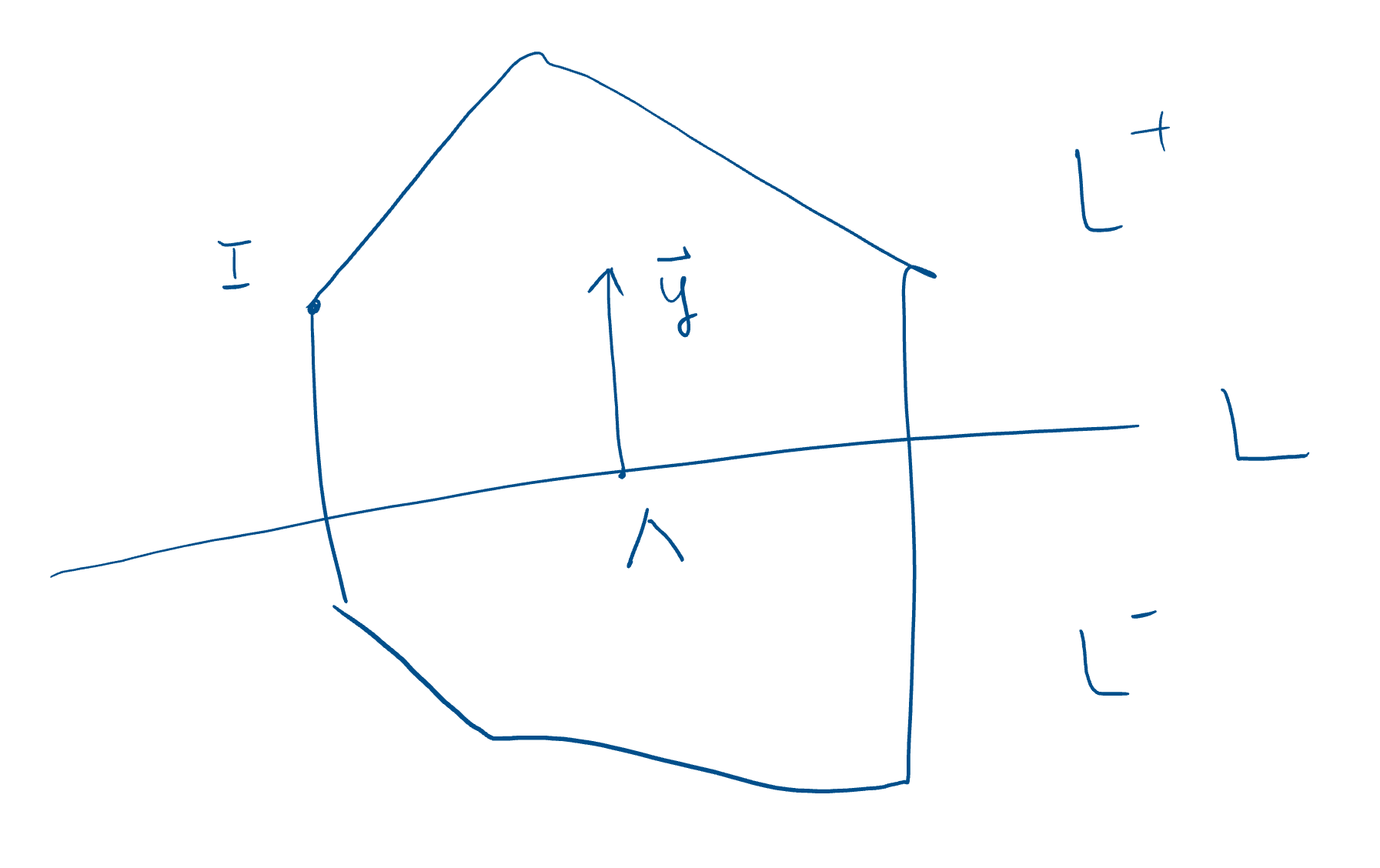# A Parameterization of Newton Polytope of a Polynomial with All Positive Coefficients

Consider a polynomial $p=\sum_{I} a_I x^I$ with $a_I\geq 0$ for all $I$ , where $x^I=\prod_{i=1}^N x_i^{n^I_i},$ its Newton polytope $N(p)$ is defined as the convex hull of vectors $\{\mathbf{n}^I=(n^I_1,\dots,n^I_N)\}$ .

Now consider the `scattering map’ $\mathbf X(x)=\frac{\partial \log p}{\partial \log \mathbf x},$ where $x_i\in (0,\infty)$ for all $i$ . We claim that $\mathbf{X}$ is an one-to-one map between $(0,\infty)^N$ and the interior of $N(p)$ .

Firstly, suppose the matrix $(n_i^I)$ does not have full rank, i.e. there exists $\mathbf s\neq 0$ such that $\mathbf s \cdot \mathbf n^I=0$ for all $I$ . In this case, $\mathbf s\cdot\mathbf X=\mathbf s\cdot\frac{\partial \log p}{\partial \log \mathbf x}=0.$ Therefore, one can project $\operatorname{im}(\mathbf X)$ by setting some $x_i=1$ so that there’s no more vector $\mathbf s$ such that $\mathbf s\cdot \mathbf n^I=0$ for all $I$ . Conversely, one can recover the original image by solving these equations $\mathbf s\cdot\mathbf X=0$ . Therefore, we can assume that the matrix $(n_i^I)$ has full rank, then $\dim (N(p))=N$ .

Next we show that interior points of $N(p)$ are in the $\operatorname{im}(\mathbf{X})$ . Let $\mathbf{\Lambda}$ be a interior point in $N(p)$ defined by $\mathbf \Lambda=\sum_{I}\lambda_I\mathbf n^I =\frac{\partial}{\partial \log \mathbf x}\sum_{I}\lambda_I \log x^I$ where $\sum_I \lambda_I=1$ and $\lambda_I > 0$ . Equations $\mathbf X(x)=\mathbf \Lambda$ are \begin{aligned} 0=\frac{\partial }{\partial \log \mathbf x}\left( \log p-\sum_{I}\lambda_I \log x^I \right)=\frac{\partial }{\partial \log \mathbf x}\left( \log F(\mathbf x) \right)=\frac{1}{F(\mathbf x)}\frac{\partial F(\mathbf x)}{\partial \log \mathbf x} \end{aligned} where $F(\mathbf x)=\sum_I a_I x^I\prod_J (x^{-J})^{\lambda_J}.$ Let $\mathbf y=\log \mathbf x$ , and then \begin{aligned} F(\mathbf y) &=\sum_I a_I \exp\left(\mathbf{y}\cdot \left(\mathbf{n}^I-\mathbf{\Lambda}\right)\right) \end{aligned} so we only need to show that $F(\mathbf y)$ has saddle points in $\mathbb R^N$ because $F>0$ for all $\mathbf y$ .

To see it, we firstly notice that $F(\mathbf y)$ is a strict convex function of $\mathbf y$ . In fact, the Hessian of $F(\mathbf y)$ is $H_{ij}(\mathbf y)=\frac{\partial^2}{\partial y_i\partial y_j}F(\mathbf y)=\sum_I a_I \exp\left(\mathbf{y}\cdot \left(\mathbf{n}^I-\mathbf{\Lambda}\right)\right)\left(\mathbf{n}^I-\mathbf{\Lambda}\right)_i\left(\mathbf{n}^I-\mathbf{\Lambda}\right)_j.$ For any $\mathbf v\neq 0$ , $\sum_{i,j}v_iv_jH_{ij}=\sum_I a_I \exp\left(\mathbf{y}\cdot \left(\mathbf{n}^I-\mathbf{\Lambda}\right)\right) \left(\mathbf v\cdot (\mathbf{n}^I-\mathbf{\Lambda})\right)^2 >0.$ It cannot vanish because that it only happens when $\mathbf v\cdot (\mathbf{n}^I-\mathbf{\Lambda})=0$ for all $I$ , but we have assumed $\{\mathbf n^I\}$ has full rank.

For a strict convex function $F(\mathbf y)$ on $\mathbb R^N$ , it has a unique minimal in $\mathbb R^N$ iff it does not take its minimal when $\mathbf{y}$ goes to infinity along any direction. In our case, we only need that for any $\mathbf{y}\neq 0$ and $\mathbf\Lambda \in (N(p))^\circ$ , there exist some $I$ such that $\mathbf{y}\cdot (\mathbf{n}^I-\mathbf{\Lambda})>0$ and if $\mathbf\Lambda \not\in N(p)$ , there exist some $\mathbf{y}\neq 0$ such that the exponentials are all negative, then $F(t\mathbf y)\to 0$ when $t\to \infty$ .In fact, if $\mathbf\Lambda \in (N(p))^\circ$ , for a given $\mathbf y\neq 0$ , the hyperplain $L$ with normal vector $\mathbf y$ crossing $\mathbf \Lambda$ divides the space $\mathbb R^N$ into semi-spaces $L^+$ and $L^-$ , then $\mathbf{n}^I$ in $L^+$ are what we are looking for. Conversely, if $\mathbf\Lambda \not\in N(p)$ , one can find a hyperplain $L$ such that $N(p)\subset L^-$ , then the normal vector of $L$ gives the wanted direction. These are direct results of convexity of $N(p)$ .

Therefore, we have proven that $F(\mathbf y)$ has a unique minimal in $\mathbb R^N$ when $\mathbf \Lambda \in (N(p))^\circ$ and no minimal in $\mathbb R^N$ when $\mathbf \Lambda \not\in N(p)$ . Hence, $\mathbf X(x)=\frac{\partial \log p}{\partial \log \mathbf x},$ is an one-to-one map between $(0,\infty)^N$ and the interior of $N(p)$ .

Generally, let $\mathbf{\Lambda}$ be a interior point in Minkowski sum $\sum_p \alpha_p N(p)$ corresponding to $P=\prod_p p^{\alpha_p}$ defined by $\mathbf{\Lambda} =\sum_p \alpha_p \mathbf{\Lambda}_p =\sum_p \alpha_p \sum_{I_p}\lambda_{I_p}\mathbf{n}^{I_p} =\frac{\partial}{\partial \log \mathbf{x}}\sum_{p}\alpha_p\sum_{I_p}\lambda_{I_p} \log x^{I_p}$ where $\sum_{I_p} \lambda_{I_p}=1$ and $\lambda_{I_p} > 0$ . Equations $\mathbf{X}(x)=\mathbf{\Lambda}$ are \begin{aligned} 0=\frac{\partial }{\partial \log \mathbf{x}}\left( \log P-\sum_{p}\alpha_p\sum_{I_p}\lambda_{I_p} \log x^{I_p} \right)&=\frac{\partial }{\partial \log \mathbf{x}}\left( \log \left(\prod_p F_p^{\alpha_p}\right) \right), \end{aligned} where \begin{aligned} F_p(\mathbf y)&=\sum_{I_p} a_{I_p} \exp\left(\mathbf{y}\cdot \left(\mathbf{n}^{I_p}-\mathbf{\Lambda}_p\right)\right). \end{aligned} Write $F(\mathbf y)=\prod_p F_p(y)^{\alpha_p},$ we only need to show that $F(y)$ has a unique saddle point in $\mathbb R^N$ .

We assume that $\alpha_p>0$ for all $p$ . Here, $F(\mathbf y)$ is no longer a strict convex function. However, take a positive number $\alpha_0$ such that $\alpha_0< \alpha_p$ for all $p$ , consider a new function $F(y)^{1/\alpha_0}=\prod_p F_p(y)^{\alpha_p/\alpha_0},$ since $F_p$ are convex functions and $\alpha_p/\alpha_0>1$ , $F_p(y)^{\alpha_p/\alpha_0}$ are convex functions, so is their product. Since $F(y)^{1/\alpha_0}$ has the same minimums as $F(y)$ , so we can further assume that $\alpha_p>1$ for all $p$ .

Finally, we claim that $F(y)$ does not take minimum when $\mathbf{y}$ goes to infinity along any direction. It is because that for a given $\mathbf{y}$ and each $p$ , $F_p(t\mathbf{y})\to \infty$ when $t\to \infty$ , so does $F(t\mathbf{y})=\prod_p F_p(t\mathbf{y})^{\alpha_p}$ . Therefore, equation $\mathbf{X}(x)=\mathbf{\Lambda}$ has a unique solution.